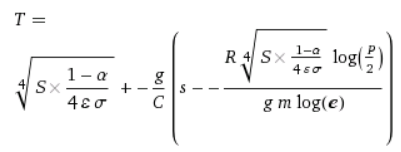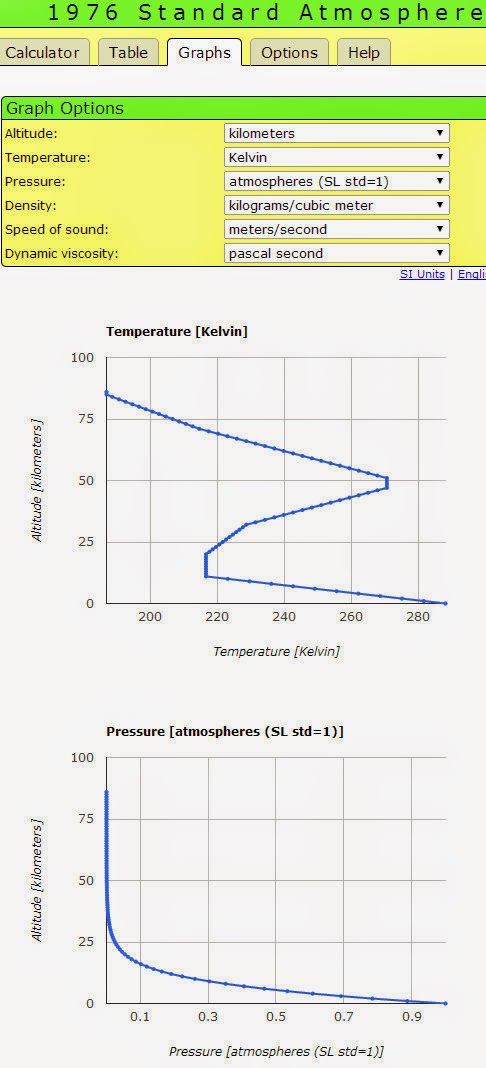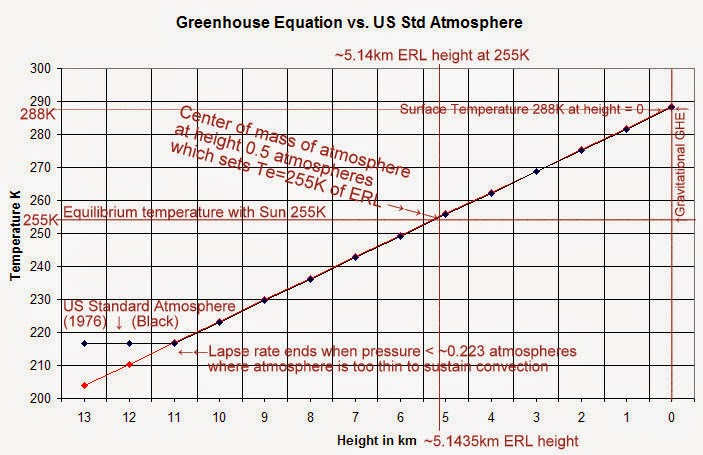## Saturday, November 29, 2014

### The Greenhouse Equation predicts temperatures within 0.28°C throughout entire troposphere without radiative forcing from greenhouse gases

In this continuing series of posts on the greenhouse equation, we will now calculate and plot the tropospheric temperature profile as a function only of the balance of radiative forcing from the Sun and gravitational forcing upon atmospheric mass, and without any contribution from greenhouse gas radiative forcing. We will see that the greenhouse equation reproduces observations and the 1976 standard atmosphere database within 0.28°C at every height from the surface all the way to the ~11,000 meter average height at the top of the troposphere.

The greenhouse equation in essence determines the temperature based upon the balance of gravitational potential energy and radiative forcing from the only source of energy, the Sun, and is unique for each height from the surface to top of the troposphere. The greenhouse equation maintains the balance of solar/radiative and gravitational/convective equilibrium, and there is one and only one temperature that maintains this balance for each height in the troposphere.

Note the only radiative forcing considered by the greenhouse equation is from the Sun, and nothing from greenhouse gases. The solar forcing warms the surface, which causes all gases to convect, rise, and expand, and which keeps the atmosphere inflated against the force of gravity, and these forces balance at every local height to maintain a horizontal local equilibrium at that particular height. Note the overall atmosphere is not in equilibrium, which is what drives this whole process of convection/adiabatic lapse rate/and the tropospheric temperature gradient.

Thus, the opposing forces of convection and gravitation at each local height are in local equilibrium. The solar radiative forcing causes all gases at the surface to warm, rise, and expand against the gravitational force which is constantly pulling all gases back to the surface. Gravitational potential energy increases the higher a gas packet rises and expands, until that gas packet reaches a height where it's temperature is the same as the surrounding air, at which the gravitational potential energy starts to exceed its kinetic energy, then the gravitational potential energy accumulated takes over to make that gas packet subside/fall/compress and warm due to the ideal gas law. Once that gas packet becomes warm enough from compression to once again overcome gravitational potential energy, it starts to rise once again and that whole process continues over and over again ad infinitum.

This rising/falling and expansion/compression of gas packets is thus driven by two opposing forces:
• Solar radiative forcing to make gases warm, rise, and expand until they cool to the same temperature as the surrounding air, followed by
• Gravitational forcing pulling the now cooled gases back to the Earth
The balance between these two opposing forces is what generates the entire 33C greenhouse effect. Radiation spectra from greenhouse gases are the result, not the cause, of the temperature gradient in the troposphere that these two dominating, very strong, and opposing forces create. (As an example, in an earlier post, we calculated that the effect of gravity on the atmosphere is creating 10,500 kilograms of gravitational forcing per square meter at the surface.)

The greenhouse equation was derived in the recent series of posts:The "Greenhouse Equation" calculates temperature (T) at any location from the surface to the top of the troposphere as a function of atmospheric mass/gravity/pressure and radiative forcing from the Sun only, and without any radiative forcing from greenhouse gases. Note the pressure (P) divided by 2 in the greenhouse equation is the pressure at the center of mass of the atmosphere (after density correction), where the temperature and height are equal to the equilibrium temperature with the Sun and ERL respectively.
and I'll now show the unique numeric solutions perfectly reproduce within 0.28C the temperature at every height in the 1976 Standard Atmosphere database from the surface all the way to ~11,000 meters height, after which the atmosphere at < ~0.223 atmospheres (units) becomes too thin to sustain convection and the equation no longer applies. This is near the transition between the troposphere and tropopause, which is where Robinson & Catling, Nature 2014, demonstrated the transition begins due to loss of convection, not greenhouse gas radiative forcing. Above the tropopause transition level (which is isothermal for several km), increased greenhouse gases cause increased cooling

The greenhouse equation solves for the single unique temperature at every height necessary to balance the vertical equilibrium of the atmosphere (since gravitational forcing is vertical only). The atmosphere is in horizontal equilibrium at every height relative to the center of mass at that coordinate. The equation applies as long as the troposphere is capable of sustaining convection, up to ~12,000km in the tropics, but beyond that the atmosphere is too thin to sustain convection and the adiabatic lapse rate, thus the equation is not applicable above this point around 11-12 km average:

We find the greenhouse equation perfectly reproduces (within 0.28C) the standard tropospheric temperature profile (black=Standard Atmosphere, red=the greenhouse equation). Here's the data calculated by Wolfram Alpha for the greenhouse equation, and the 1976 Standard Atmosphere data:

 height (km) Greenhouse Equation T in K Std Atmos 1976 T in K 0 288.433 288.15 1 281.933 281.65 2 275.433 275.15 3 268.933 268.65 4 262.433 262.15 5 255.933 255.65 6 249.433 249.15 7 242.933 242.65 8 236.433 236.15 9 229.933 229.65 10 223.433 223.15 11 216.933 216.65 12 210.433 216.65 13 203.933 216.65

1976 Standard Atmosphere:(Note the greenhouse equation does correct for the change in density with pressure, and the natural logarithmic decay of pressure with altitude)

We annotate the plot which shows the height of the "effective radiating level" or ERL, the height at which the local horizontal equilibrium at that particular height must by definition be where T = equilibrium temperature with the Sun = 255K, at essentially exactly at the center of mass of the atmosphere, which has to coincide with the location where half of the mass is above and half below, where the pressure is one-half the surface pressure after altitude and density correction - both of which are done by the greenhouse equation (and is the reason for the natural logarithms in the equation):There is nothing in the greenhouse equation which is dependent upon radiative forcing from greenhouse gases, nor greenhouse gas concentrations, nor greenhouse gas absorption/emission spectra, and yet we can perfectly reproduce the temperature within 0.28C anywhere in the troposphere and at the surface. Thus, the absorption and emissions of IR from greenhouse gases are the consequence, and not the cause of the real 33C greenhouse effect.

Here are some of the numerical solutions from Wolfram Alpha which did the for the one and only unique value T that satisfies the greenhouse equation horizontal equilibrium at a particular height (s) in kilometers above the surface, which is the one and only variable that I changed for each of the these numeric solutions, as you can see from the input and Wolfram Alpha solutions:

Try it yourself: Here's the code for the greenhouse equation to determine the T at height = 0 (i.e. at the surface). I have bolded that 0 in the code below so you can find it. Simply change that variable from 0 to whatever height in kilometers from the surface to calculate the one and only unique local equilibrium at that particular height solution for the greenhouse equation temperature gradient:

T = [1367 (1 - 0.3) / (4*1* (5.6704*10^-8))]^1/4 +((-6.5)*(0-[-[8.31*{[1367 (1 - 0.3) / (4*1* (5.6704*10^-8))]^(1/4)}*log(1/2)]/[9.8*0.029*log(e)]/1000]))

1.You still have not come to grips with the most basic issue: the difference between incoming and outgoing radiative power.

The earth and its atmosphere absorb about 240 W/m^2 from solar radiation, averaged over time and area (so 240 * Aearth watts). We have good measurements on this, well within +/-10 W/m^2.

The earth's surface emits about 390 W/m^2 of infrared radiation, again averaged over time and area (so 390 *Aearth watts). We have good measurements on this as well, also well within +/-10 W/m^2.

Nobody, alarmist or skeptic, thinks the earth is more than about 1.0 W/m^2 out of balance. So how do we make up this 150 W/m^2 imbalance?

You seem to think this comes from the weight of the atmosphere in earth's gravitational field. This weight is a physical force. For a force to transfer energy it must act while moving over a distance (Work = Force * Distance). The power at any instant from a mechanical force is Force * Velocity (using the components of these vectors in the same direction).

We use this in hydroelectrice power to exploit the force of falling water and its resultant velocity through turbines.

But the atmosphere has already fallen as far as it can go. So it cannot provide power to the the surface through this mechanism.

Through all your math you have not dealt with this basic energy imbalance. In your analysis, how do you rectify this?

1.The entire greenhouse equation is derived from

1st Law of Thermodynamics and the very first assumption made is Ein=Eout
Ideal Gas Law
Newton's Second Law of Motion F=ma which becomes F=mg

That's it.

There are far too many steps to prove to you at every point E is conserved, and that radiative forcing is never introduced. Radiance is a function of T, which is a function of mass/gravity/pressure forcing plus radiative forcing from the only power source the Sun.

The radiative GHE gets this all backwards.

The fact that the equation reproduces the observed average temperature of the troposphere from the surface to ~11,000 meters offers overwhelming evidence this theory is correct.

You can't have it both ways - only one of the theories can be correct, otherwise the Earth would be 66K warmer rather than 33K warmer.

2."We have good measurements on this as well, also well within +/-10 W/m^2.""

This is widely believed, but not by scientists at NASA who have estimated the rate of net energy imbalance to be 0.5 Wm-2 with errors an order of magnitude greater (17 Wm-2)

You can read a summery of the NASA work here with references and links to the papers:

https://geoscienceenvironment.wordpress.com/2014/09/04/the-emperors-of-climate-alarmism-wear-no-clothes/

2.Darn it,

I have set up the GH equation in MS Excel and I am getting 288.45K at s = 0km and 281.95 at s = 1km.

I know this is only a slight difference, but I can't see where it might come from.

Just computer error?.. seems too large

-------------
AndyG55

1.rounding errors, I assume on the part of Excel since Wolfram has a much better reputation for math software, but who knows?

In any event, that's only a tiny 0.017C difference, negligible

What version Excel are you using?

3.2007, I'll check on 2010 at work tomorrow

AndyG55

4.AndyG55, set the TSI as 1361 and the deviations from the ISA disappear.
-wayne